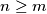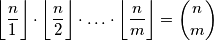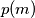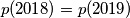### MEMO 2018 pojedinačno problem 4

Kvaliteta:
Avg: 0,0
Težina:
Avg: 6,0

a) Prove that for very positive integer$m$ there exists an integer$n \geq m$ such thatb) Denote by$p(m)$ the smallest integer$n \geq m$ such that the above equation holds. Prove$p(2018) = p(2019)$.

Izvor: Srednjoeuropska matematička olimpijada 2016, pojedinačno natjecanje, problem 4